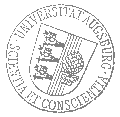Universität AugsburgInstitut für MathematikAugsburger Mathematisches Kolloquium

Dr. Daniel Egger
IBM Zürich

spricht am

Dienstag, 14. Mai 2019

um

17:30 Uhr

im

Raum 2004 (L1)

über das Thema:

»Quantum applications for finance«

 Abstract: We present a quantum algorithm that analyzes risk more efficiently than Monte Carlo simulations traditionally used on classical computers. We employ quantum amplitude estimation to price securities and evaluate risk measures such as Value at Risk and Conditional Value at Risk on a gate-based quantum computer. Additionally, we show how to implement this algorithm and how to trade off the convergence rate of the algorithm and the circuit depth. The shortest possible circuit depth - growing polynomially in the number of qubits representing the uncertainty - leads to a convergence rate of \$O(M^{-2/3})\$ where \$M\$ is the number of samples. This is already faster than classical Monte Carlo simulations which converge at a rate of \$O(M^{-1/2})\$. If we allow the circuit depth to grow faster, but still polynomially, the convergence rate quickly approaches the optimum of \$O(M^{-1})\$. Thus, for slowly increasing circuit depths our algorithm provides a near quadratic speed-up compared to Monte Carlo methods. We demonstrate our algorithm using two toy models. In the first model we use real hardware, such as the IBM Q Experience, to price a Treasury-bill (T-bill) faced by a possible interest rate increase. In the second model, we simulate our algorithm to illustrate how a quantum computer can determine financial risk for a two-asset portfolio made up of government debt with different maturity dates. Both models confirm the improved convergence rate over Monte Carlo methods. Using simulations, we also evaluate the impact of cross-talk and energy relaxation errors.

 Hierzu ergeht herzliche Einladung. Prof. Dr. Maxim Smirnov

Kaffee, Tee und Gebäck eine halbe Stunde vor Vortragsbeginn im Raum 2006 (L1).

[Impressum]      [Datenschutz]      wwwadm@math.uni-augsburg.de,     Mo 15-Apr-2019 10:30:03 MESZ